#### How to Calculate EMI in Excel?

With these simple steps, we are going to learn how to calculate the EMI. The EMI is the Equated Monthly Installment. It is easy to calculate the EMI in Excel. It can be used to calculate the EMI of your mortgage, car loan, or any other loan.

It is important to calculate the EMI to be able to manage your household budget. The lower the monthly installment, the more money you have for other expenses.

## How to calculate EMI?

Layout the information you already know, without EMI calculation.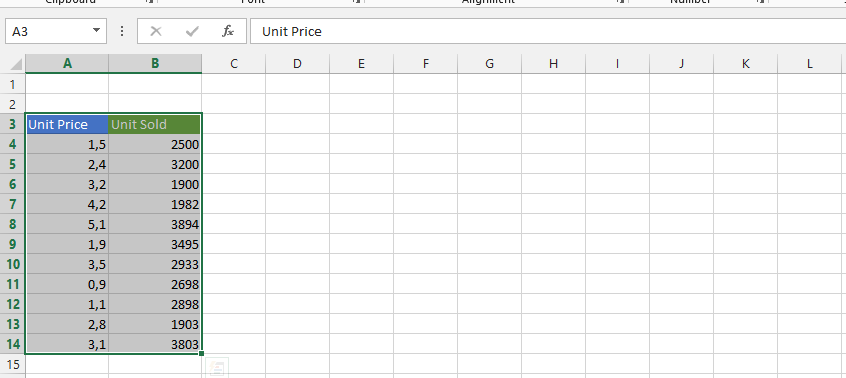Click on the column that is beside the monthly installment and type in =PMT(rate,nper,pv,[fv],[type])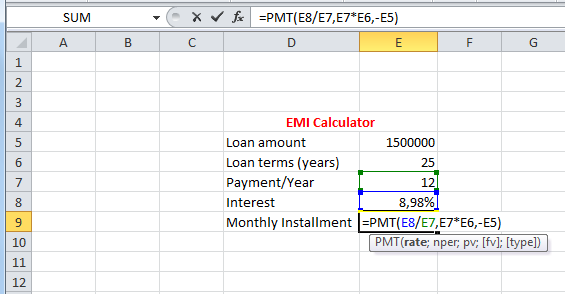=PMT(E8/E7,E7*E6,-E5) is the formula here.

Note:

• The rate is interest divided by the payment per year,
• nper is loan terms multiplied by the count of payments,
• pv was the loan amount with a minus symbol in front. The minus symbol means that this is the money you own.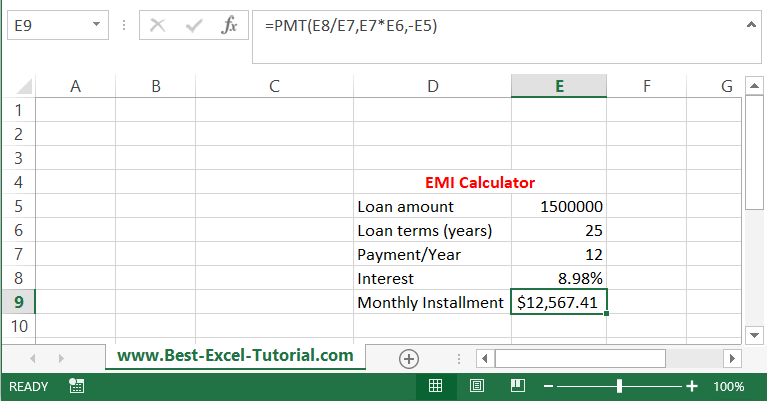This is the result. Your monthly installment will be \$12,567.41. This is the calculated EMI for \$1,5m for 25 years with an annual interest rate of of 8.98%.

## An alternative method using RATE

There is another method to calculate EMI in Excel using the RATE function. Here are the steps to calculate EMI using the RATE function:

1. Enter the loan amount in one cell. If the loan amount is 1,00,000, enter it in cell A1.
2. Enter the loan tenure in the next cell. If the loan tenure is 3 years, enter it in cell A2.
3. Enter the number of payments per year in the next cell. If the payments are monthly, enter 12 in cell A3.
4. Enter the interest rate per annum in the next cell. If the interest rate is 10%, enter it in cell A4.
5. In the cell where you want to display the EMI, type the following formula:

=-(A1RATE(A2A3,-A2*A3,A4/12))

By using this formula, you can easily calculate the EMI in Excel using the RATE function.

## How to calculate the total loan cost and total interest based on EMI?

But what is the total cost of such a loan? Let’s calculate the total loan costs and total interest in such a situation.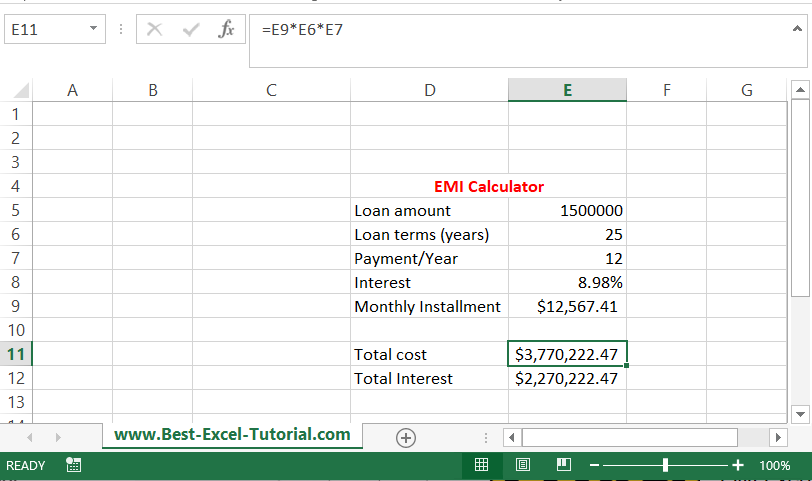The total cost is EMI * years * months. The formula is =E9*E6*E5 in my example.

Total interest is just the total cost-loan amount (=E11-E5).

The total cost of such a loan would be over \$3,77m and the total interest over \$2,27m.

## How to calculate the loan amount based on a given EMI?

This is how to calculate EMI with Excel. What if you know your target EMI and would like to know the loan amount you will be able to borrow?

Go to the Data Tab on the Ribbon and click on What-If Analysis and choose Goal Seek.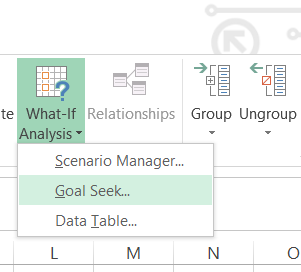A new dialog window appears. Let’s assume you can afford a \$15,000 monthly installment. Goal Seek parameters will be:

• Set cell: the cell containing your EMI (E9 in this case).
• To value: we agreed on a value of \$15,000.
• By changing cell: the cell with the loan amount (E5 here).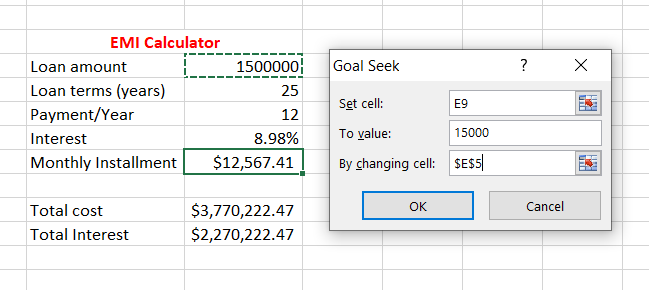A new loan amount is calculated.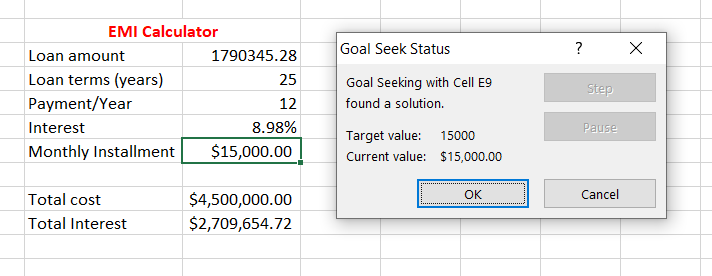You can borrow \$1,790,345.28 under the given loan conditions and assume a monthly payment of \$15,000.

## Scenario Analysis

Excel allows you to perform scenario analysis by changing variables such as interest rates, loan tenures, or loan amounts to see how they affect your EMI. This is a powerful tool for decision-making because it enables you to evaluate different loan scenarios and their impact on your budget.

You can create data tables or use Excel’s built-in Scenario Manager to compare various loan options side by side.

## Understanding Amortization

EMI calculations inherently involve amortization, which is the process of reducing the loan principal over time. By studying the amortization schedule, you gain insights into how much of each EMI payment goes toward interest and how much toward principal.

This understanding can motivate you to make extra payments toward the principal to reduce the total interest paid and shorten the loan tenure.

## Extra Payments and Prepayment

Excel is a valuable tool for exploring the impact of making extra payments or prepaying your loan. By adjusting the EMI formula to account for extra payments, you can determine how much time and interest you can save.

Excel can help you create a prepayment schedule and visualize the benefits of early loan repayment.

## Sensitivity Analysis

Sensitivity analysis is a technique that involves changing multiple variables simultaneously to assess how they collectively impact your EMI. This approach helps you understand the complex interplay between factors like interest rates, loan amounts, and tenures.

Excel’s What-If Analysis tools, including Data Tables and Goal Seek, can assist you in performing sensitivity analysis to make well-informed financial decisions.

In this lesson, you learned:

• Why is it so important to calculate EMI?
• How to calculate EMI?
• How to calculate total amount?
• How to calculate total loan costs and total interest costs?

Ultimately, mastering EMI calculations in Excel empowers you to take control of your financial future. You can plan your budget effectively, make informed borrowing decisions, and align your financial goals with your loan commitments. Excel’s flexibility and analytical capabilities put you in the driver’s seat, ensuring that you’re making financial choices that serve your best interests.